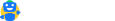# Section 50 – Capital Gain on Sale of Depreciable Assets

Updated on:

08 min read

If the person sells a capital asset that forms part of the block of assets on which depreciation has been allowed as per the provisions of the Income Tax Act, the income from such sales is a capital gain.

The calculation of capital gain or loss arising on the sale of depreciable assets can be divided into two categories:

• Where some assets are sold from the blocks of assets.
• Where all assets of the block are transferred. And the block of assets ceases to exist.

## Calculation of capital gain where part of the block of assets is transferred:

Situation I

1. When net sale consideration on such asset’s sale is reduced from the written down value (opening WDV + cost of assets acquired if any) of the block of the assets.
2. And the written down value of the block of the asset becomes Nil (Note: The written down value can be Nil but not negative).
3. Then the income from such transfer of capital asset shall be considered to be a short-term capital gain.

Computation of short-term capital gain:

 Sale consideration xxx Less Opening written down value of the block xxx Less Actual cost of any asset acquired during the financial year xxx Short-term capital gain xxx

Situation II

1. When net sale consideration on such asset’s sale is reduced from the written down value (opening WDV + cost of assets acquired if any) of the block of the assets.
2. And the written down value of the block of asset is not Nil.
3. There is no capital gain on transfer of assets.
4. Hence, normal depreciation will be allowed.

## Calculation of capital gain where all the assets of the block are transferred:

Situation III

1. If the whole of the block of asset is sold and the sale consideration is less than the written down value (opening WDV + cost of assets acquired if any) of the block of assets.
2. Then there is short-term capital loss on sale of block of asset.
3. And no depreciation shall be allowed from such block of assets.

Computation of short-term capital loss:

 Opening written down value of the block xxx Add Actual cost of the asset acquired xxx Less Sale consideration xxx Short-term capital loss xxx

Situation IV

1. If whole of the block of assets is sold and the sale consideration is more than the written down value (opening WDV + cost of assets acquired if any) of the block of assets.
2. Then income from such sale of block of asset is short-term capital gain.

Computation of short-term capital gain:

 Sale consideration xxx Less Opening written down value of the block xxx Less Actual cost of the asset acquired xxx Short-term capital gain xxx

Note: Capital gain or loss on the transfer of depreciable assets is always treated as short-term capital gain or loss.File your income tax for FREE in 7 minutes
Free, simple and accurate. Designed by tax expertsHave a query?
mailto@cleartax.in
Company
Individuals
Tax Experts
SMEs
Enterprises
ITR Resources
MF Resources
Tools
ClearGST
ClearServices